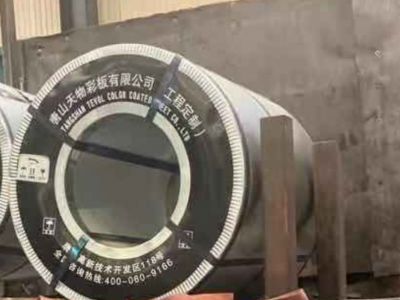# 你怎么知道彩涂板镀层涂层有多厚？

## 你怎么知道彩涂板镀层涂层有多厚？（1） 纯镀锌板镀层厚度=双面镀层重量÷2÷7.3，如镀层重量为180g/m2，镀层厚度=180÷2÷7.3=12.32um；
（2） 含铝15%的镀锌板镀层厚度=双面镀层重量÷2÷6.8，如镀层重量为150g/m2，镀层厚度=150÷2÷6.8=11.03μm；
（3） 55%镀铝锌板的镀层厚度=双面镀层重量÷2÷5.2，如镀层重量为150g/m2，镀层厚度=150÷2÷5.2=14.42μM。
2。锌层重量计算

（1） 镀锌板镀层重量=镀层厚度×7.3×2，如试验厚度为12.32，镀层重量为12.32×7.3×2=180g/m2；
（2） 含铝15%的镀锌板镀层重量=镀层厚度×6.8×2，如试验厚度为11.03，则镀层重量为11.03×6.8×2=150g/m2；
（3） 55%铝锌板镀层重量=镀层厚度×5.2×2。例如，如果试验厚度为14.42，涂层重量为14.42×5.2×2=150g/m2。

Users who pay attention to the quality of color coated plate will have some requirements for the aluminum zinc content and coating of color coated plate, but as users, how to measure and calculate the weight of coating and the thickness of coating and coating?

First of all, we have to have the most basic testing tool: magnetic coating thickness gauge
1. Zinc layer thickness measurement
First, use salt and bagel cloth to remove the coating on the surface of the color plate (the technique should be delicate to prevent damage to the coating), then use the magnetic thickness gauge to measure the thickness of the coating, measure three points on the same plate, and take the average value.
The thickness of the coating can also be roughly calculated according to the weight of each square meter of double-sided coating provided by the material sheet or label. The specific calculation formula is as follows:
(1) Coating thickness of pure galvanized sheet = weight of double-sided coating ÷ 2 ÷ 7.3, for example, if coating weight is 180g / m2, coating thickness = 180 ÷ 2 ÷ 7.3 = 12.32um;
(2) Coating thickness of galvanized sheet containing 15% aluminum = weight of double-sided coating ÷ 2 ÷ 6.8, for example, if the coating weight is 150 g / m2, coating thickness = 150 ÷ 2 ÷ 6.8 = 11.03 μ m;
(3) The coating thickness of 55% aluminized zinc plate = weight of double-sided coating ÷ 2 ÷ 5.2, for example, if the coating weight is 150 g / m2, the coating thickness = 150 ÷ 2 ÷ 5.2 = 14.42 μ M.
2. Weight calculation of zinc layer
The weight of the coating can be calculated by the thickness of the coating measured by the magnetic thickness gauge. The specific calculation formula is as follows:
(1) Coating weight of galvanized sheet = coating thickness × 7.3 × 2, for example, if the test thickness is 12.32, the coating weight is 12.32 × 7.3 × 2 = 180g / m2;
(2) The coating weight of galvanized sheet containing 15% aluminum = coating thickness × 6.8 × 2, for example, if the test thickness is 11.03, the coating weight is 11.03 × 6.8 × 2 = 150g / m2;
(3) The coating weight of 55% aluminum zinc plate = coating thickness × 5.2 × 2. For example, if the test thickness is 14.42, the coating weight is 14.42 × 5.2 × 2 = 150 g / m2.
3. Coating thickness measurement
After the thickness of the coating is detected, the total thickness of the coating and the coating is detected by the same method. Three points are measured on the same plate surface, and the average value is taken.
The thickness of coating can be calculated by subtracting the total thickness of coating. For example, the total thickness of coating and coating measured by magnetic thickness meter is 36 μ m, while the coating thickness is 11 μ m, so the coating thickness is 36-11 = 25 μ M.
【相关推荐】

400-060-9166
• 400电话

400-060-9166